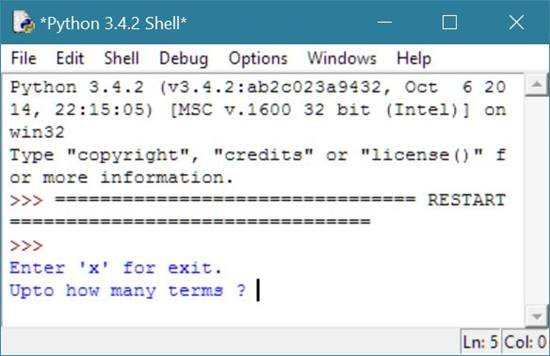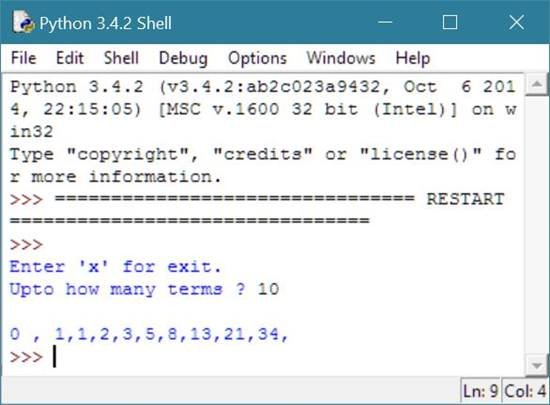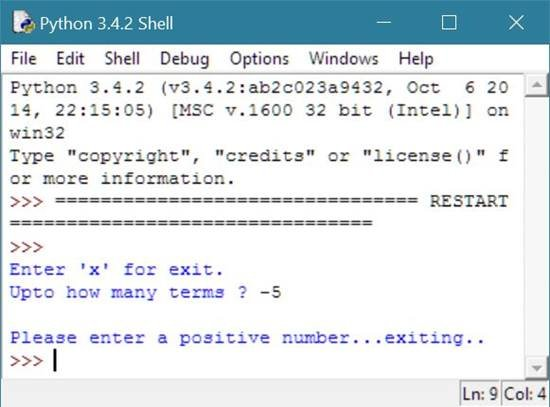# Python Program to Print Fibonacci Series

## Print Fibonacci Series in Python

To print fibonacci series in python, you have to ask from user to enter the limit or to enter the total number of term to print the fibonacci series upto the given term.

As we know that the Fibonacci series starts from 0 and 1, and after that, every next number is the summation of the last two number. Therefore to print fibonacci series in python, just print 0 and 1 if the given limit is greater than 2 and then apply the summation of the previous two numbers to print the next number as shown in the program given below.

## Python Programming Code to Print Fibonacci Series

Following python program ask from user to enter the limit upto which he/she want to print the Fibonacci series to print Fibonacci series upto to the given term:

```# Python Program - Print Fibonacci Series

print("Enter 'x' for exit.");
terms = input("Upto how many terms ? ");
if terms == 'x':
exit();
else:
term = int(terms);
a = 0;
b = 1;
count = 2;
print();
if term == 0:
elif term < 0:
elif term == 1:
print(a);
else:
print(a,",",b,end=",");
while count < term:
c = a + b;
print(c,end=",");
a = b;
b = c;
count = count + 1;
```

Here is the sample run of the above python program to illustrates how to print Fibonacci series:

Below is the initial output asking to enter any number (that will be the limitation) of total fibonacci series to print:Now enter the term say 10 to print total 10 number in fibonacci series as shown in sample output given below:If user supplied a negative number and press enter then here is the sample output you will see:Below is the same program directly written and run on python shell:### Same Program in Other Languages

You may also like to learn or practice the same program in other popular programming languages:

Tools
Calculator